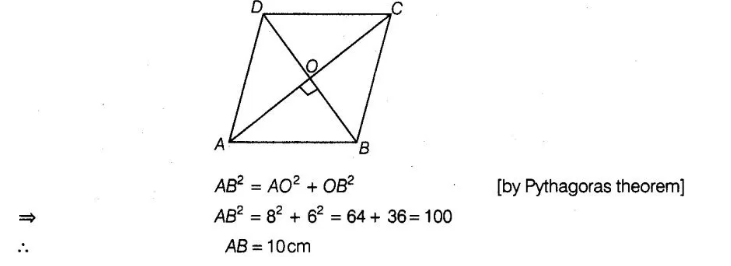# If the lengths of the diagonals of

Question:

If the lengths of the diagonals of rhombus are 16 cm and 12 cm. Then, the length of the sides of the rhombus is

(a) 9 cm

(b) 10 cm

(c) 8 cm

(d) 20 cm

Solution:

(b) We know that, the diagonals of a rhombus are perpendicular bisector of each other.

Given,                             AC = 16 cm and BD = 12 cm                                                [let]

∴                                       AO = 8cm, SO = 6cm

and                           ∠AOB = 90°

In right angled ∠AOB,